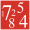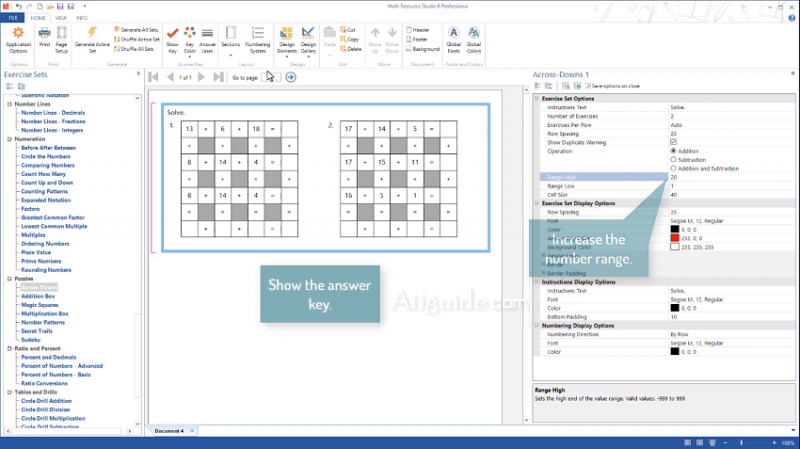#### Math Resource Studio - Related Programs# Math Resource Studio7.0.173

• Evaluate:
• Operating system:
Windows 7, 8, 10
• Language: English
• Version: 7.0.173
• Latest updated:
• Publisher: Schoolhouse Technologies
• File size: 40.5 Mb

## Download Math Resource Studio 7 for Windows 7, 8, 10 - Generate individual or class sets of math worksheets, workbooks, or tests quickly and effortlesslyMath Resource Studio 7 is a useful tool which allows teachers and other adults to create mathematics workshops. As exams can be tailored around the needs of the individual student or an entire class, this program is extremely flexible. One of the main reasons why such an alternative may be chosen is that it cuts down on paper-based waste while providing a host of different customization options that might not otherwise be possible.

Math Resource Studio makes it easy to create differentiated math worksheets to target the learning needs of all of your students. Generate worksheets with the exact practice required to advance skills to the next level.

Producing math practice worksheets with Math Resource Studio is as simple as selecting the types of questions you want, setting a few options, and clicking the generate button to create a completely original math worksheet. A total of more than 120 unique math worksheet activities in all. And with the many optional settings for each activity, there are literally hundreds of activity configurations available.

## Overview of Math Resource Studio Features:

• Advanced addition, subtraction, multiplication, multiplication with powers of ten, division, division with remainders, division with no remainders, mixed operations, multiple addends, and multiple operations.
• Basic addition, doubles addition, addition with regrouping control, division, multiplication, subtraction, subtraction with regrouping control, fact families, input output, make sums, match-ups, mixed operations, pictorial addition, quick facts, word problems with addition, subtraction, multiplication, and division.
• Addition, subtraction, multiplication, and division with fractions, comparing fractions, division with whole numbers, fraction identification with grids and rectangles, fractions and decimals, mixed numbers and improper fractions, mixed operations with fractions, multiplication and division with whole numbers, equivalent fractions, and simplifying fractions.
• Solving equations with defined variables, equations with single variable, inequalities, number problems, and pre-algebra.
• Producing math practice worksheets with Math Resource Studio is as simple as selecting the types of questions you want and how many, setting a few options to control the difficulty of the questions, and clicking the generate button.
• Easily create the exact math worksheets you require to support your instructional objectives and desired learning outcomes.
• and more...
Comment:
Write a comment
Top alternative apps [Freeware]:
Top alternative apps [Shareware]: